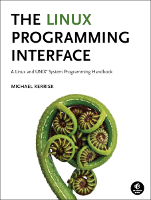altio/poll_pipes.c

This is altio/poll_pipes.c (Listing 63-2, page 1340), an example from the book, The Linux Programming Interface.

This page shows the "distribution" or "book" version of the file (why are there two versions?), or the differences between the two versions. You can switch between the views using the tabs below.

In the listing below, the names of Linux system calls and C library functions are hyperlinked to manual pages from the Linux man-pages project, and the names of functions implemented in the book are hyperlinked to the implementations of those functions.Function list (Bold in this list means a function is not static)

/* poll_pipes.c

Example of the use of poll() to monitor multiple file descriptors.

Usage: poll_pipes num-pipes [num-writes]
def = 1

Create 'num-pipes' pipes, and perform 'num-writes' writes to
randomly selected pipes. Then use poll() to inspect the read ends
of the pipes to see which pipes are readable.
*/
#include <time.h>
#include <poll.h>
#include "tlpi_hdr.h"
int
main(int argc, char *argv[])
{
int numPipes, ready, randPipe, numWrites, j;
struct pollfd *pollFd;
int (*pfds);                     /* File descriptors for all pipes */

if (argc < 2 || strcmp(argv, "--help") == 0)
usageErr("%s num-pipes [num-writes]\n", argv);

/* Allocate the arrays that we use. The arrays are sized according
to the number of pipes specified on command line */

numPipes = getInt(argv, GN_GT_0, "num-pipes");
numWrites = (argc > 2) ? getInt(argv, GN_GT_0, "num-writes") : 1;

pfds = calloc(numPipes, sizeof(int ));
if (pfds == NULL)
errExit("calloc");
pollFd = calloc(numPipes, sizeof(struct pollfd));
if (pollFd == NULL)
errExit("calloc");

/* Create the number of pipes specified on command line */

for (j = 0; j < numPipes; j++)
if (pipe(pfds[j]) == -1)
errExit("pipe %d", j);

/* Perform specified number of writes to random pipes */

srandom((int) time(NULL));
for (j = 0; j < numWrites; j++) {
randPipe = random() % numPipes;
printf("Writing to fd: %3d (read fd: %3d)\n",
pfds[randPipe], pfds[randPipe]);
if (write(pfds[randPipe], "a", 1) == -1)
errExit("write %d", pfds[randPipe]);
}

/* Build the file descriptor list to be supplied to poll(). This list
is set to contain the file descriptors for the read ends of all of
the pipes. */

for (j = 0; j < numPipes; j++) {
pollFd[j].fd = pfds[j];
pollFd[j].events = POLLIN;
}

errExit("poll");